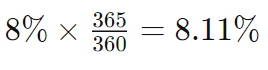What Is Bank Discount Basis?# What Is Bank Discount Basis?

4 Min.

The bank discount bias, also known as discount yield, is a measure of the expected return of a bond sold at a price lower than its face value or par value. It is predominantly used to determine the yield on treasury bills, commercial paper, and municipal notes. These bonds are purchased at a discounted price, held until maturity, and then sold at face value, resulting in a profit for the holders. The bank discount bias is an annualized yield expressed as a percentage. It is calculated using a 30-day month and a 360-day year.

## Basics

Discount yield, commonly referred to as bank discount basis, serves as the standard approach employed by financial entities in pricing discounted fixed-income securities like municipal and U.S. Treasury bills. The quotation is expressed as a percentage of the face value and is calculated by applying a 360-day-count convention, assuming twelve 30-day months in a year.

## Exploring Bank Discount Basis

The bank discount basis represents an annualized percentage yield, denoting the return on investment achieved through the acquisition of an instrument at a discount and subsequent sale at par upon bond maturity. Treasury bills, as well as various corporate commercial paper and municipal notes, are commonly issued at a discount from their par value.

U.S. Treasury bills possess a maximum maturity of 52 weeks, with Treasury notes and bonds featuring more extended maturity dates. Despite the prevalence of the 30/360 day-count convention in quoting treasury bonds, it's crucial to note that the bank discount rate, due to its consideration of 365 days in a year, will yield a lower figure than the actual return on short-term money market investments. Therefore, it should not be employed as an exact measure of the anticipated yield.

Overextended maturities, the impact of the day count convention on a bond's current "price" becomes more pronounced compared to shorter maturities. To convert a 360-day yield to a 365-day yield, a simple "gross-up" can be applied using the factor 365/360. For instance, an 8% 360-day yield would translate to an 8.11% yield based on a 365-day year.## How the Bank Discount Rate Is Calculated

Deriving the bank discount rate involves employing the following formula:

where:

• DPV = Discount from par value
• PV = Par value
• PP = Purchase price​

For instance, let's consider an investor acquiring a \$10,000 Treasury bill at a \$300 discount from its par value, resulting in a purchase price of \$9,700. Assuming a maturity period of 120 days, the discount yield can be calculated as follows:

or a 9% yield.

## Discount Yield vs. Bond Accretion

In the evaluation of securities sold at a discount, the discount yield method is employed to determine the investor's rate of return, presenting a distinct approach from bond accretion. Bonds utilizing bond accretion may be issued at par, at a discount, or a premium. The accretion process redistributes the discount amount into bond income throughout the remaining bond life.

Consider an investor acquiring a \$1,000 corporate bond for \$920, maturing in 10 years. Upon maturity, the investor receives \$1,000, with the \$80 discount constituting bond income, complemented by the bond's accrued interest.

In contrast, bond accretion entails allocating the \$80 discount to bond income over the bond's 10-year lifespan. The investor can opt for either the straight-line method, distributing a consistent annual amount into bond income, or the effective interest rate method, a more intricate formula for calculating bond income. Bonds with coupons may also be quoted on a yield basis.

## Conclusion

The bank discount basis, or discount yield, serves as a crucial metric for assessing the expected return on bonds sold at a price below their face value. Widely applied in the pricing of fixed-income securities, such as treasury bills and municipal notes, this annualized percentage yield is calculated using a 30-day month and a 360-day year. The method involves purchasing bonds at a discounted price, holding them until maturity, and selling them at face value, generating profits for investors. While exploring the intricacies of the bank discount basis, it becomes evident that the 30/360 day-count convention and considerations for the actual return on short-term investments contribute to a nuanced understanding of this financial metric. In essence, the bank discount rate provides a valuable tool for investors navigating the complexities of the bond market.

Bank Discount Basis
Treasury Bill
Commercial Paper
Municipal Note
Bond Accretion
Discount Yield
Products
Contacts